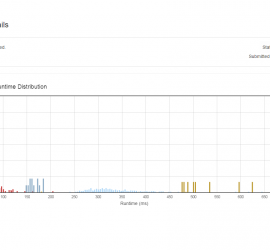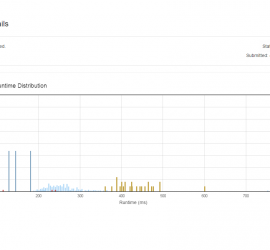# Daily Archives: June 3, 2015

## [leetcode] Decode Ways

Decode Ways A message containing letters from A-Z is being encoded to numbers using the following mapping: ‘A’ -> 1 ‘B’ -> 2 … ‘Z’ -> 26 Given an encoded message containing digits, determine the total number of ways to decode it. For example, Given encoded message “12”, it could […]## [leetcode] Subsets II

Subsets II Given a collection of integers that might contain duplicates, nums, return all possible subsets. Note: Elements in a subset must be in non-descending order. The solution set must not contain duplicate subsets. For example, If nums = [1,2,2], a solution is: [ , , [1,2,2], [2,2], [1,2], [] […]## [leetcode] Gray Code

Gray Code The gray code is a binary numeral system where two successive values differ in only one bit. Given a non-negative integer n representing the total number of bits in the code, print the sequence of gray code. A gray code sequence must begin with 0. For example, given […]